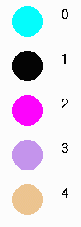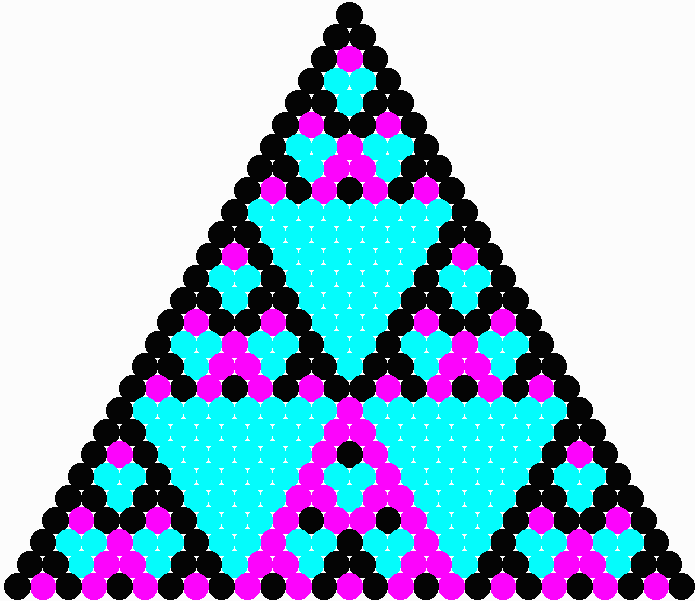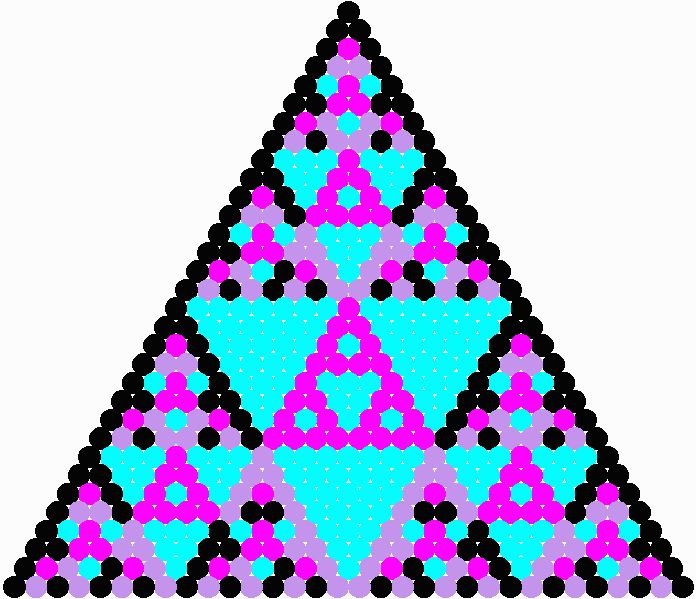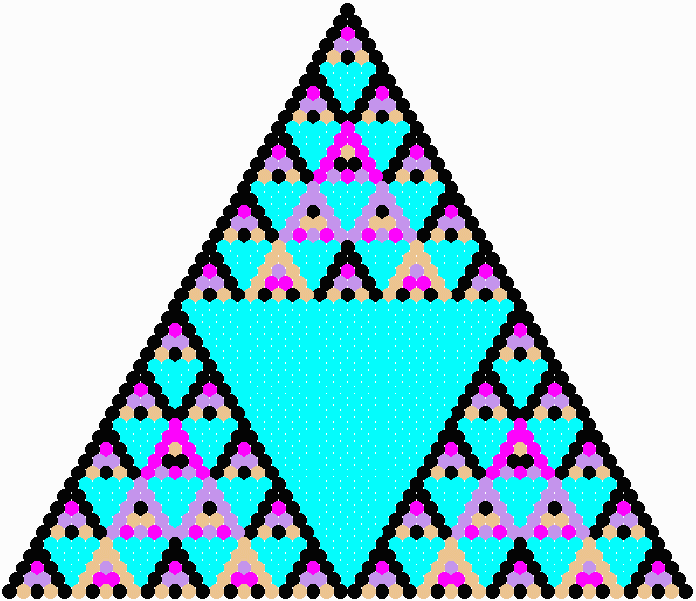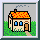The Twist
Basically, Pascal's Triangle ( symmetric version) is generated by starting with ones down the sides and generating the inside entries so that each entry is the sum of the two entries directly above and to the left and directly above and to the right. Suppose that instead of using regular addition to generate the interior entries, you used modular arithmetic (also known as clock arithmetic). You may or may not remember seeing modular arithmetic in school, but you use it regularly when you deal with time. Three hours after 11:00 it is not 14:00 but rather 2:00. You add three to eleven but when you get to twelve you start over again at 0. Modular arithmetic works the same way, except that, like the military, we use 0 and we don't use the last number (you never hear them talking about 2400 hours, right?). We also can do modular arithmetic with numbers other than 12 for the maximum. For example, we can use five as the maximum, in which case we call it mod five arithmetic. In mod five arithmetic, we use the numbers 0, 1, 2, 3, and 4, and three plus three is one, while two plus two is still four. If we do arithmetic mod three, two plus two is one but one plus one is still two, etc. You can see the addition tables for a number of different mod numbers by following this link. One nice thing about modular arithmetic is that there are only a finite number of possible answers. If you assign to each of the possible answers a color, then the triangle can be presented as an array of colored dots, or circles. Most of us find looking for patterns in this kind of image more appealing than looking for patterns in the numbers themselves. Below are the first few rows of Pascal's Triangle using mod 3, mod 4 and mod 5 addition, expressed as arrays of colored circles. For these red is 0, black is 1, green is 2, blue is 3 and yellow is 4.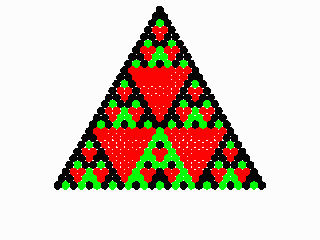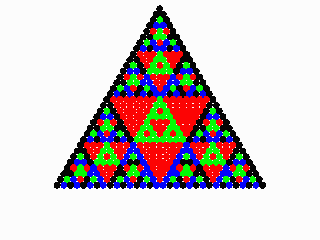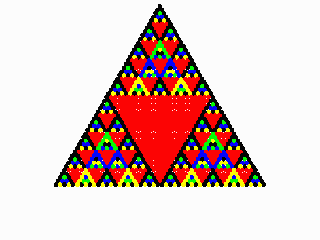Pascal's Triangle Mod 3 27 rows Pascal's Triangle Mod 4 32 rows Pascal's Triangle Mod 5 50 rows
Sometimes we can see things differently if we use different colors. Here are the same patterns with different color assignments: